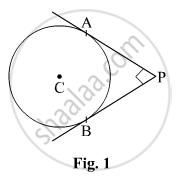Share
Notifications

View all notifications
Books Shortlist
Your shortlist is empty

# In Fig. 1, Pa and Pb Are Two Tangents Drawn from an External Point P to a Circle with Centre C and Radius 4 Cm. If Pa ⊥ Pb, Then the Length of Each Tangent Is: - CBSE Class 10 - Mathematics

Login
Create free account

Forgot password?
ConceptCircles Examples and Solutions

#### Question

In Fig. 1, PA and PB are two tangents drawn from an external point P to a circle with centre C and radius 4 cm. If PA ⊥ PB, then the length of each tangent is:• (A) 3 cm

• (B) 4 cm

• (C) 5 cm

• (D) 6 cm

#### Solution

Since APbotPB,CA botAP,CBbotBP

and AC = CB = radius of the circle, therefore APBC is a square having side equal to 4 cm.

Therefore, length of each tangent is 4 cm.

Is there an error in this question or solution?

#### Video TutorialsVIEW ALL 

Solution In Fig. 1, Pa and Pb Are Two Tangents Drawn from an External Point P to a Circle with Centre C and Radius 4 Cm. If Pa ⊥ Pb, Then the Length of Each Tangent Is: Concept: Circles Examples and Solutions.
S